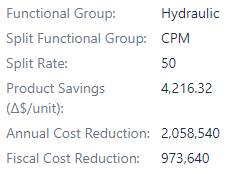# Cumulative sum of two different fields that has the same options

Good morning,
I would like to help to solve this issue.

I have two fields, one named as “Functional Group” and the other “Split Functional Group”. Both have the same options to choose. Above I have a “Split Rate” and “Fiscal Cost Reduction”.I already have a sum chart that shows me the fiscal cost reduction per functional group along time. However, now I need another that shows me:
If I don’t have a “Split Functional Group” and “Split Rate” filled, then the Functional Group has 100% of “Fiscal Cost Reduction”. But if I have, the “Split Rate” shows the % of the division. The first one will take 50% of the value and the other the rest.
But I need a graph that: if I select “CPM” (one option available in “Functional Group” and “Split Functional Group”), it will show me the sum of both fields: when it is the main and when it is the split.

What I have today:
Cumulative sum of “Functional Group”, already considering when have “Split Rate” or not.
([Measures].[Split Rate with due date]/100)*([Measures].[Fiscal Cost Reduction with due date on Maturity], [Functional Group].DefaultMember)

Cumulative sum of “Split Functional Group”.
((100-[Measures].[Split Rate with due date])/100)*([Measures].[Fiscal Cost Reduction with due date on Maturity], [Split Functional Group].DefaultMember)
Both together is the dashed line.
The problem: how can I create a filter to select one option (“CPM”) and show me the sum of both graphs?

I tried to write as explained as possible, but I’m available to explain more if necessary.

Thank you very much!!!

I cannot put “CPM” in both filters, because “Functional Group” and “Split Functional Group” always have different options (not make sense if both are equal)

I hope I got our request correctly. You would like to use the Split rate only in case a particular Split Functional group is selected in the report. If this is the use case, I would suggest using issue-level calculation for Split functional group selection and use a simple formula for All member selection in Split functional group.

Issue level calculation is needed to get correct values for percentage calculation on total value when both percentage values and total value is set on issues.

Here is a formula example. It calculates the value per due date. You can apply the cumulative calculation if need on this.

``````CASE WHEN
[Split Functional Group].CurrentMember.Level.Name = "Split Functional Group"
THEN
-- no split functional group is selected then 100% split rate no calculation is needed
[Measures].[Fiscal Cost Reduction with due date on Maturity]
ELSE
-- split functional group is selected then apply split rate per each issue individually
Sum(Filter(
Descendants([Issue].CurrentMember,[Issue].[Issue]),
DateInPeriod(
[Measures].[Issue due date],
[Time].CurrentHierarchyMember
)),
[Measures].[Split Rate with due date]/100
*
[Measures].[Fiscal Cost Reduction with due date on Maturity]
)
END
``````

The description:

indicates some rules based on Split Functional group only. Therefore, I did not add any specific use case for Functional Group. The formula should work for any selection of Functional group. Please share more details if I miss here something.

Daina / support@eazybi.com